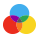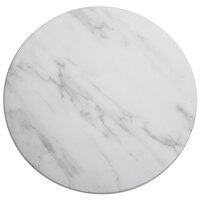ColorsFrom $58.74/Each Reg.Lots of 6$61.49$58.74 From$31.30/Each

Reg.Lots of 8
$32.99$31.30

From $21.19/Each Reg.Lots of 6$21.99$21.19$25.99/Each

From $23.02/Each Reg.Lots of 6$23.99\$23.02